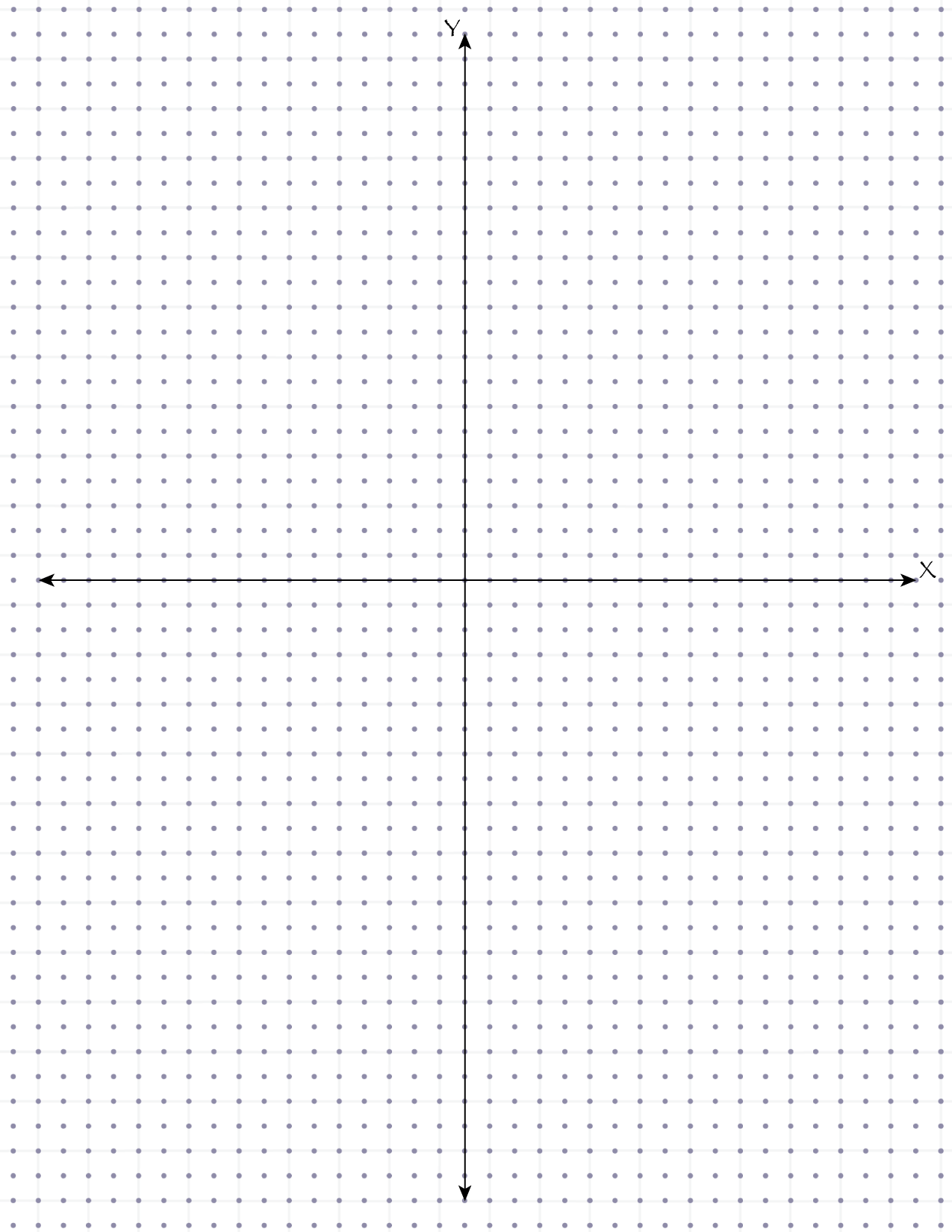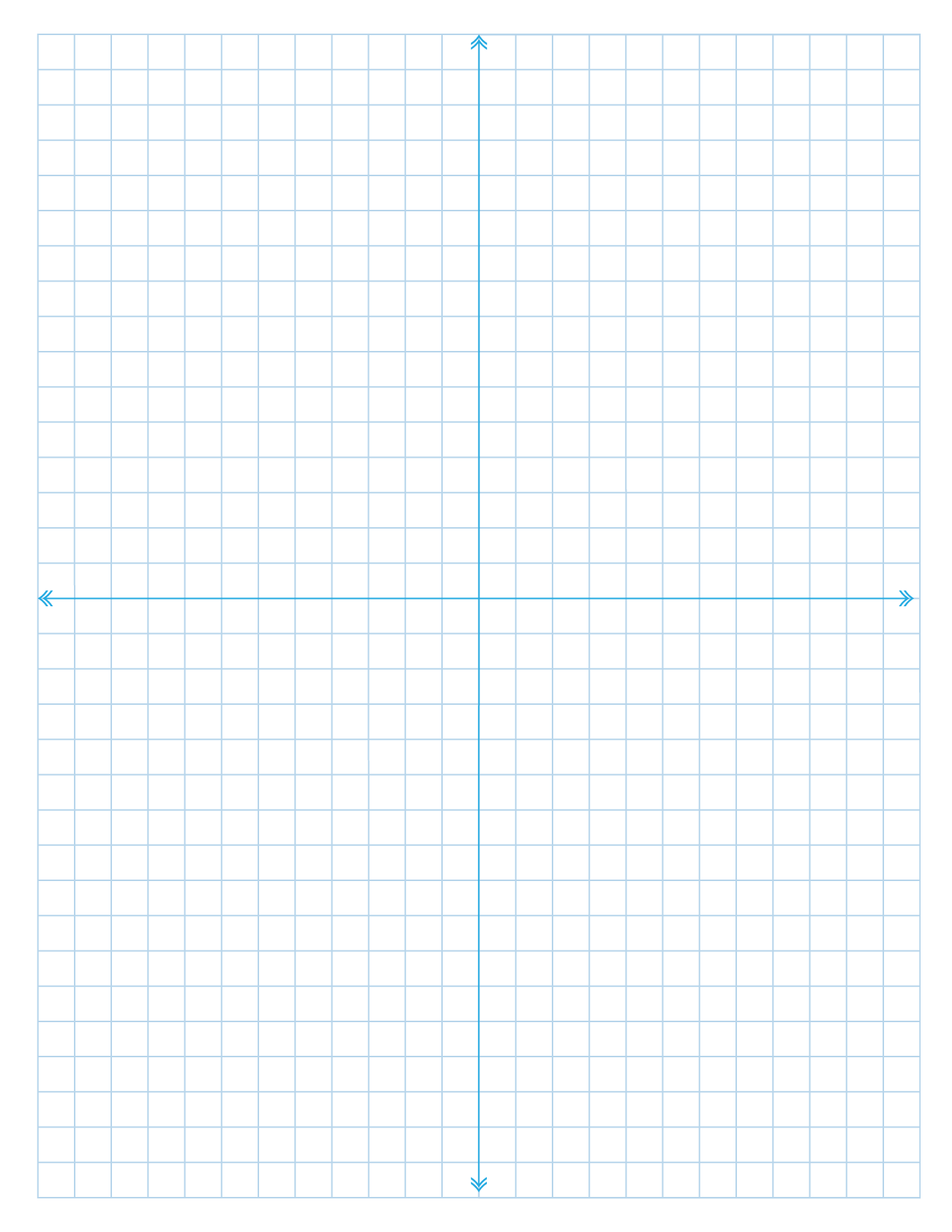Graph paper is used to plot different points for mathematical equations (Khan Academy has a great video on how to find each point on the graph). While you can draw the graph yourself, it makes it so much easier to have one that you can just print out!Traditional graph paper has an X axis and a Y axis. This refers to the two lines you see on the image above. The X axis runs left to right, and the Y axis runs top to bottom.To tell someone where a point is in the graph, you write its location (X, Y). This gives the number of spaces from the center on the X axis, and the number of spaces from the center on the Y axis. The point is located at the intersection of those values.Each quarter (or quadrant) of the graph has a positive or negative X value, and a positive or negative Y value. Many simple equations only deal with the top right quadrant, which is where all values are positive.

Top Right: (+, +)

Top Left: (-, +)

Bottom Right: (+, -)

Bottom Left: (-,-)